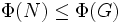# Frattini subgroup is normal-monotone

This article describes a fact or result that is not basic but it still well-established and standard. The fact may involve terms that are themselves non-basic
View other semi-basic facts in group theory
VIEW FACTS USING THIS: directly | directly or indirectly, upto two steps | directly or indirectly, upto three steps|
This fact is an application of the following pivotal fact/result/idea: characteristic of normal implies normal
View other applications of characteristic of normal implies normal OR Read a survey article on applying characteristic of normal implies normal

## Statement

### Verbal statement

The Frattini subgroup of any normal subgroup is contained in the Frattini subgroup of the whole group, provided the normal subgroup is a group in which every proper subgroup is contained in a maximal subgroup.

(Note that this group property is always satisfied when the normal subgroup is a finite group, so for finite groups, the Frattini subgroup of a normal subgroup is always contained in the Frattini subgroup of the whole group).

### Statement with symbols

Let$N$ be a normal subgroup of a group$G$, where$N$ satisfies the property that every proper subgroup is contained in a maximal subgroup. Then,$\Phi(N)$, the Frattini subgroup of$N$, is contained in$\Phi(G)$, the Frattini subgroup of$G$.

### Property-theoretic statement

The subgroup-defining function that sends a group to its Frattini subgroup is a normal-monotone subgroup-defining function (with some assumptions on the nature of the groups).

## Definitions

### Frattini subgroup

The Frattini subgroup of a group is the intersection of all its maximal subgroups. In other words, an element is in the Frattini subgroup if it is in every maximal subgroup.

## Proof

### Proof outline

The proof uses four facts (for convenience, we denote the group by$G$ and normal subgroup by$N$):

1. Characteristic subgroups of normal subgroups are normal: This fact helps us show that the Frattini subgroup of the subgroup$N$, is in fact normal in the whole group$G$
2. For a group where every proper subgroup is contained in a maximal subgroup, the Frattini subgroup is a Frattini-embedded normal subgroup: a normal subgroup whose product with any proper subgroup is proper. Thus$\Phi(N)$ is a Frattini-embedded normal subgroup inside$N$.
3. Frattini-embedded normal in subgroup and normal implies Frattini-embedded normal: This allows us to show that the Frattini subgroup of the subgroup, is Frattini-embedded normal inside the whole group. In other words,$\Phi(N)$ is a Frattini-embedded normal subgroup inside$G$
4. For any group, any Frattini-embedded normal subgroup is contained inside the Frattini subgroup: This allows us to conclude that the Frattini subgroup of

### Hands-on proof

Given: A group$G$,a normal subgroup$N$ of$G$ with the property that any proper subgroup of$N$ is contained in a maximal subgroup

To prove:$\Phi(N) \le \Phi(G)$

Proof: The Frattini subgroup$\Phi(N)$ is a characteristic subgroup of$N$. Since every characteristic subgroup of a normal subgroup is normal,$\Phi(N)$ is a normal subgroup of$G$.

We need to show that$\Phi(N)$ is contained in$\Phi(G)$. For this, it suffices to show that$\Phi(N)$ is contained in every maximal subgroup of$G$. We prove this by contradiction.

Suppose$M$ is a maximal subgroup of$G$ not containing$\Phi(N)$. Then, the subgroup generated by$M$ and$\Phi(N)$ is the whole of$G$. Since$\Phi(N)$ is normal in$G$,$M\Phi(N) = G$. From that, it follows that$\Phi(N)(M \cap N) = N$ (this is a particular example of the modular property of groups).

Since$M$ does not contain$\Phi(N)$,$M$ does not contain$N$ either and hence$M \cap N$ is a proper subgroup of$N$. Since every proper subgroup is contained in a maximal subgroup of$N$, there is a maximal subgroup of$N$ containing both$M \cap N$ and$\Phi(N)$ . This contradicts the fact that their product is$N$.

Normality of$N$ is thus crucial because it guarantees normality of$\Phi(N)$. This in turn is crucial in converting a subgroup-generated statement to a product of subgroups statement.